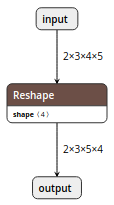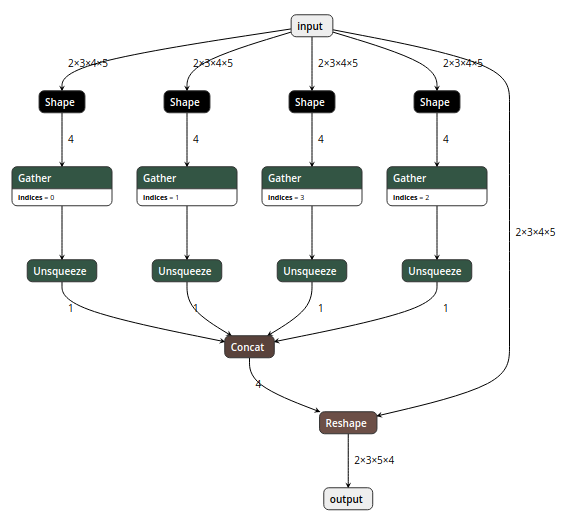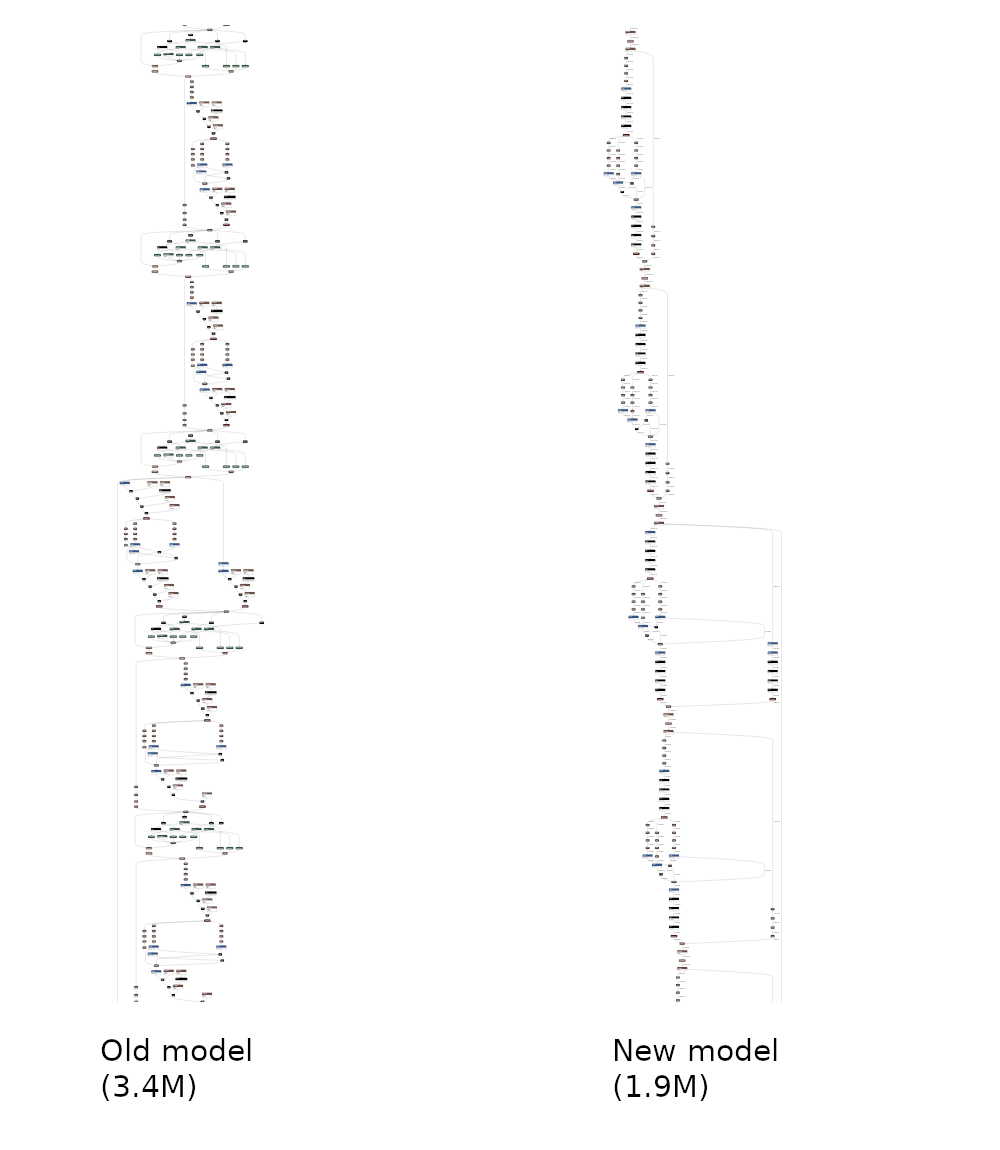# sergregory / onnx-simplifier

Simplify your onnx model

Geek Repo

Github PK Tool

# ONNX Simplifier

ONNX is great, but sometimes too complicated.

## Background

One day I wanted to export the following simple reshape operation to ONNX:

```import torch

class JustReshape(torch.nn.Module):
def __init__(self):
super(JustReshape, self).__init__()

def forward(self, x):
return x.view((x.shape, x.shape, x.shape, x.shape))

net = JustReshape()
model_name = 'just_reshape.onnx'
dummy_input = torch.randn(2, 3, 4, 5)
torch.onnx.export(net, dummy_input, model_name, input_names=['input'], output_names=['output'])```

The input shape in this model is static, so what I expected isHowever, I got the following complicated model even after polishing:Moreover, there are also some operations performed on weights (like this), which can all be eliminated by offline computation.

## Our solution

ONNX Simplifier is presented to simplify the ONNX model. It infers the whole computation graph and then replaces the redundant operators with their constant outputs.

### Web version

We have published ONNX Simplifier on https://convertmodel.com. It works out of the box and doesn't need any installation. Just open the webpage, choose ONNX as the output format, check the onnx simplifier and then select your model to simplify.

### Python version

If the web version doesn't work well for you, you can install the Python version via pip (with python > 3.5):

``````pip3 install onnx-simplifier
``````

Then

``````python3 -m onnxsim input_onnx_model output_onnx_model
``````

For more functions like skipping optimization and setting input shape manually (when input shape is dynamic itself), try the following command for help message

``````python3 -m onnxsim -h
``````

## Demonstration

An overall comparison between a complicated model and its simplified version:## In-script workflow

If you would like to embed ONNX simplifier python package in another script, it is just that simple.

```import onnx
from onnxsim import simplify

model = onnx.load(path + model_name + '.onnx')

# convert model
model_simp, check = simplify(model)

assert check, "Simplified ONNX model could not be validated"

# use model_simp as a standard ONNX model object```

You can see more details of the API in onnxsim/__main__.py